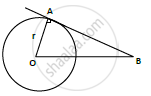Share

# O is the Center of a Circle of Radius 8cm. the Tangent at a Point a on the Circle Cuts a Line Through O at B Such that Ab = 15 Cm. Find Ob - CBSE Class 10 - Mathematics

ConceptCircles Examples and Solutions

#### Question

O is the center of a circle of radius 8cm. The tangent at a point A on the circle cuts a line through O at B such that AB = 15 cm. Find OB

#### Solution

Consider a circle with center O and radius OA = 8cm = r, AB = 15 cm.(AB) tangent is drawn at A (point of contact)

At point of contact, we know that radius and tangent are perpendicular.

In ΔOAB, ∠OAB = 90°, By Pythagoras theorem

𝑂𝐵2 = 𝑂𝐴2 + 𝐴𝐵2

OB = sqrt(8^2 + 15^2)

=sqrt(64+225)

= sqrt(289)

= 17 cm

∴ 𝑂𝐵 = 17 𝑐𝑚

Is there an error in this question or solution?

#### Video TutorialsVIEW ALL 

Solution O is the Center of a Circle of Radius 8cm. the Tangent at a Point a on the Circle Cuts a Line Through O at B Such that Ab = 15 Cm. Find Ob Concept: Circles Examples and Solutions.
S